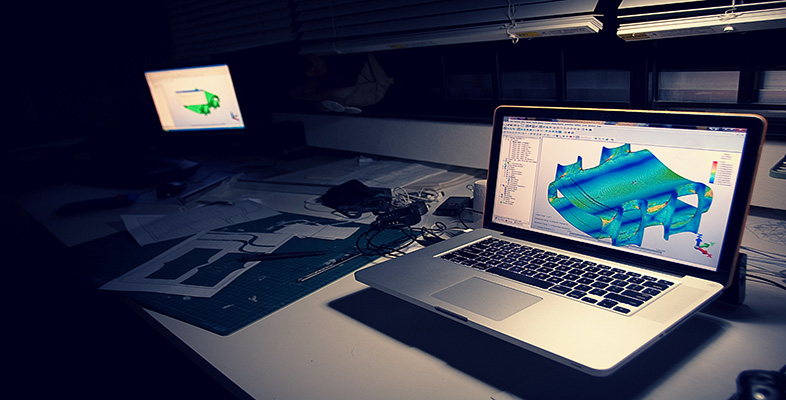Science, Maths & Technology

### Become an OU studentIntroduction to finite element analysis

Start this free course now. Just create an account and sign in. Enrol and complete the course for a free statement of participation or digital badge if available.

# Interactive step-by-step solution

1. Set title and preferences

Give your job a title, e.g. ‘Cantilever Beam’.

2. Define element types and options

Select a 2d elastic beam element.

Is this a good element choice? You can also look at the options for this element type.

3. Define material properties

Set Young’s modulus to 2.× 10 5 (in units of N/mm 2 ) and Poisson’s ratio to 0.3

4. Define beam section parameters
• set 50 for width and 100 for height.

Note that the vertical axis here is the z -axis, so the force will be applied in the z direction.

It is also a good idea to preview the section data summary to check that all the parameters are entered and calculated correctly.

The parameters of interest are:

Area = B × H = 5000

Second moment of area about y -axis

= I yy = B ×( H 3 )/12 = 0.41667× 10 7

5. Create 2 Keypoints

Create two key points at:

KP 1 = 0, 0, 0

KP 2 = 2000, 0, 0

6. Create line

Create a line between these two key points.

7. Set global element edge size

Set global element size to 200

8. Mesh the line with a default mesh

Mesh the line.

9. Apply displacement constraints

Fix all dofs at key point (or node) number 1

Apply a force of 10000 N in the minus Z direction on the node at the other end of the beam.

11. Rotate the axes

If necessary, rotate the axes so that the z-axis is pointing up:

12. Solve with default criteria

Solve the system.

13. Plot deformed shape

What is the maximum displacement at the tip?

I got 32.0 mm

14. List nodal displacement values

Here is the list of displacements I obtained as a function of node x-position:

 Node number Node x-position Displacement (Uz) 1 0 0.000 3 200 0.4637 4 400 1.7914 5 600 3.8872 6 800 6.6549 7 1000 9.9986 8 1200 13.822 9 1400 18.030 10 1600 22.526 11 1800 27.213 2 2000 31.997

You can see that the maximum displacement is 32 mm (to 2 dp).

1. List stresses in the beam

To look at the stresses in the beam we normally need to define an element table. You should read your FEA software’s help menu, particularly on your chosen element to determine the name (or identifier) of variables that give bending stresses.

I obtained the following values of axial and bending stresses for each element:

 Element number (from constrained end) Axial stress Bending stress (stresses in both nodes are computed to be the same) 1 0.00 -228.0 2 0.00 -204.0 3 0.00 -180.0 4 0.00 -156.0 5 0.00 -132.0 6 0.00 -108.0 7 0.00 -84.00 8 0.00 -60.00 9 0.00 -36.00 10 0.00 -12.00
1. Validate the bending stresses

Now you need to use your knowledge in beam theory to verify the results of your FE model. Follow the procedure below:

1. Draw a free body diagram of your beam and calculate the bending moment at the ends of each element.
2. Calculate the second moment of area about y-axis, I yy = B ×( H 3 )/12
3. Calculate the maximum bending stress at the ends of each element using the classic Engineer’s Bending Equation,

For example, for element 1 you should get bending stresses of 240 MPa at node I and 216 MPa at node J which gives the average stress of 228 MPa for element 1. This is exactly what we achieved from your finite element model.

2. Exit the program.